Courses

# Test: IUPAC Nomenclature Of Coordination Compounds

## 25 Questions MCQ Test Chemistry for JEE | Test: IUPAC Nomenclature Of Coordination Compounds

Description
This mock test of Test: IUPAC Nomenclature Of Coordination Compounds for JEE helps you for every JEE entrance exam. This contains 25 Multiple Choice Questions for JEE Test: IUPAC Nomenclature Of Coordination Compounds (mcq) to study with solutions a complete question bank. The solved questions answers in this Test: IUPAC Nomenclature Of Coordination Compounds quiz give you a good mix of easy questions and tough questions. JEE students definitely take this Test: IUPAC Nomenclature Of Coordination Compounds exercise for a better result in the exam. You can find other Test: IUPAC Nomenclature Of Coordination Compounds extra questions, long questions & short questions for JEE on EduRev as well by searching above.
QUESTION: 1

### Direction (Q. Nos. 1-10) This section contains 10 multiple choice questions. Each question has four choices (a), (b), (c) and (d), out of which ONLY ONE is correct. Q. According to IUPAC nomenclature, sodium nitroprusside is named as

Solution:

IUPAC name of sodium nitroprusside Na2[Fe(CN)5NO] is sodium pentacyanonitrosyl ferrate (III) because in it NO is neutral ligand and the oxidation number of Fe is +3. Which is calculated as Na2[Fe(CN)5NO]
2×(+1)+x+5×(−1)+1×0 = 0
2 + x - 5 = 0
x - 3 = 0
x = + 3

QUESTION: 2

### The formula of the complex hexamminecobalt (III) chloride sulphate is

Solution:

The formula of complex hexamminecobalt (III) chloride sulphate is [Co(NH3)6]CISO4.

QUESTION: 3

### The IUPAC name of the compound [Cr(NH3)5(NCS)][ZnCI4] is

Solution:

The lUPAC name of [Cr(NH3)5(NCS)][ZnCI4] is pentammine isothiocyanatochromium (III) tetrachlorozincate (II).

QUESTION: 4

The IUPAC name of the complex [(CO)5Mn - Mn(CO)5] is

Solution:

Decacarbonyldimanganese (0), Mn2(CO)10, is made up of two square pyramidal Mn(CO)5 units joined by a Mn-Mn bond.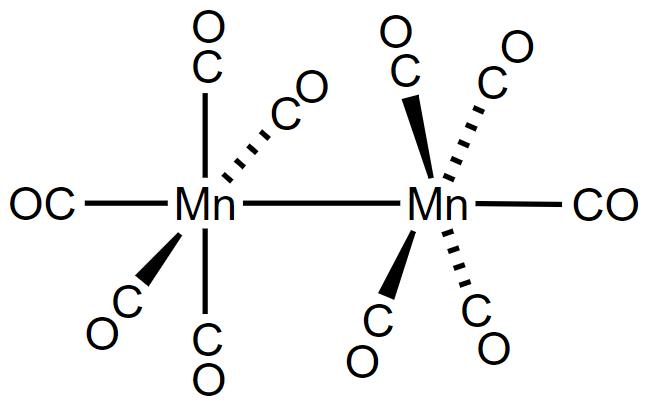QUESTION: 5

The donor sites of EDTA ligand are

Solution:

Ethylenediaminetetraacetate ion (EDTA)4- is an important hexadentate ligand. It can bind through two nitrogen and four oxygen atoms to a central metal ion.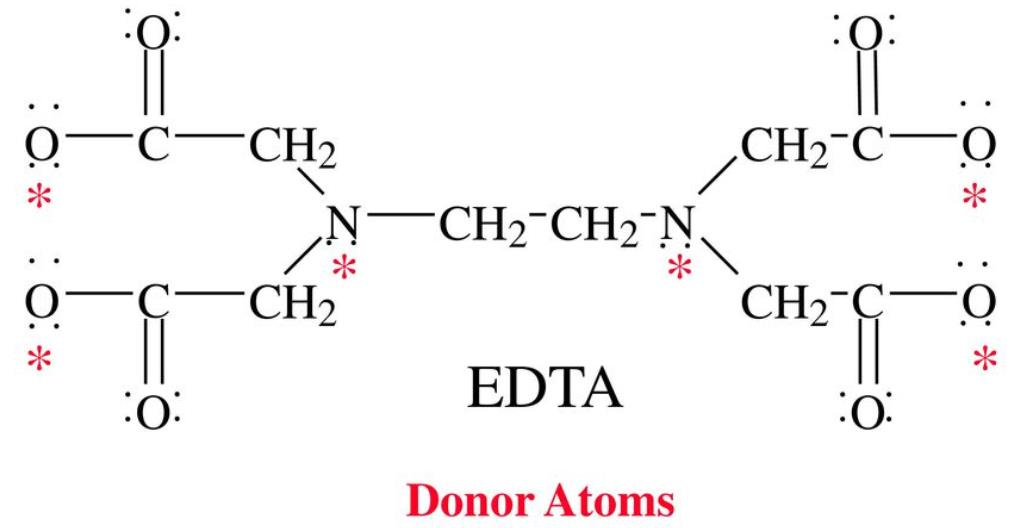QUESTION: 6

The IUPAC name of [Ni(PPh3)2CI2]2+ is

Solution:

The IUPAC name of [Ni(PPh3)CI2]2+ is dichloro bis (triphenylphosphine) nickel (ll)ion.

QUESTION: 7

Formula of brown ring complex is

Solution:

The brown ring test for nitrates depend on the ability of Fe2+ to reduce nitrates to nitric oxide, which reacts with Fe2+ to form a brown coloured complex.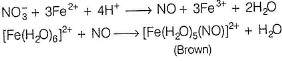QUESTION: 8

The IUPAC name of the compound K[SbCl5Ph] is

Solution:

The IUPAC name of the compound K[SbCl5Ph] is potassium pentachloro(phenyl) antimonate (V).

QUESTION: 9

K2[OsCI5N] is named as

Solution:

K2[OsCI5N] is named as potassium pentachlorozoosmate (VI)

QUESTION: 10

What is the IUPAC name of compound NaBH4?

Solution:

The IUPAC name of NaBH4 is sodium tetrahydridoborate

*Multiple options can be correct
QUESTION: 11

Direction (Q. Nos. 11-15) This section contains 5 multiple choice questions. Each question has four choices (a), (b), (c) and (d), out of which ONE or MORE THAN ONE are correct.

Q.

Which of the following complexes have correct name?

Solution:

Complexes K[Pt(NH3)Cl5] and [Ag(CN)2]- have been given their respective IUPAC names.

*Multiple options can be correct
QUESTION: 12

Which is/are the correctly named?

Solution:

DMG (bidentate and neutral) is not named correctly, acac, bipy and gly have correct names respectively.

*Multiple options can be correct
QUESTION: 13

The correct name of ligand(s) in IUPAC name is/are

Solution:

The correct name of ligand in IUPAC name are thiosulphate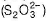and cyclopentadienyl [(C5H5)-].

QUESTION: 14

The ionisation isomer of [Cr(H2O)4Cl(NO2)Cl] is

Solution:

Ionization isomers are identical except that a ligand has exchanged places with anion or neutral molecule that was originally outside the coordination complex.
Thus, in Ionization isomerism the central ion and the other ligands are identical.
So, according to the definition, Clis replaced by NO2- in the ionization sphere.
[Cr(H2O)4Cl(NO2)Cl] ---->  [Cr(H2O)4Cl(NO2)]+ + Cl-
[Cr(H2O)4Cl2]NO2 -----> [Cr(H2O)4Cl2]+ + NO2-

*Multiple options can be correct
QUESTION: 15

The ligand(s) which gives chelate complexes is/are

Solution:

When a di or polydentate ligand used its two or more d onor atoms to bind a single metal ion. It is said to be a chelate ligand. The number of such ligating groups is called the denticity of the ligand. Such complexes are called chelate complex tend to be more stable than similar complexes containing unidentate ligands.

QUESTION: 16

Comprehension Type

Direction (Q. Nos. 16 and 17) This section contains a paragraph, describing theory, experiments, data, etc. Two questions have been related to the paragraph have been given. Each question has only one correct answer among the four given options (a), (b), (c) and (d).

Passage

In naming complexes, cation is named first followed by anion. Non-ionic or neutral complexes are given a one word name. The cationic and neutral complex ions are named by writing ‘the number and name of the ligands followed by the name of the central metal ion with its oxidation number in roman numerals within the parenthesis. If the complex is anionic, name of the metals ends with ‘ate’. When the complex contain two or more metal atoms linked with the help of bridging ligand then each bridging ligand is started with μ.

Q.

Name of [CoBr(NH3)4 NO2] is

Solution:

The correct name of [CoBr(NH3)4NO2] is tetramminebromonitrocobalt (II).

QUESTION: 17

In naming complexes, cation is named first followed by anion. Non-ionic or neutral complexes are given a one word name. The cationic and neutral complex ions are named by writing ‘the number and name of the ligands followed by the name of the central metal ion with its oxidation number in roman numerals within the parenthesis. If the complex is anionic, name of the metals ends with ‘ate’. When the complex contain two or more metal atoms linked with the help of bridging ligand then each bridging ligand is started with μ.

Q.

The IUPAC name of [Ni(NH3)4] [NiCI4] is

Solution:

The IUPAC name of [Ni(NH3)4][NiCI4] is tetramminenickel (II) tetrachloronickelate (II).

QUESTION: 18

Matching List Type

Direction (Q. Nos. 18 and 19) Choices for the correct combination of elements from Column I and Column II are given as options (a), (b), (c) and (d), out of which one is correct.

Q.

Match the Column I with Column II and mark the correct option from the codes given below.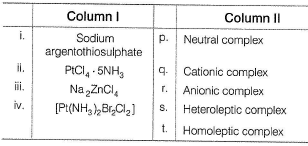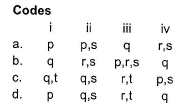Solution:

(i) → (q,t), (ii) → (q ,s), (iii) → (r,t), (iv) → (p ,s)

The complex PtCl4.5NH3 is designated as [Pt(NH3)5Cl]Cl3 which ionizes to [Pt(NH3)5Cl]3+ + 3Cl- ions. Thus, three moles of AgCl are produced from 3Cl- ions with AgNO3.
So, PtCl4.5NH3 is Cationic Complex.

QUESTION: 19

Match the Column I with Column II and mark the correct option from the codes given below.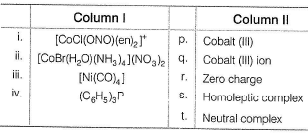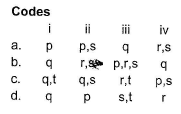Solution:

(i) → (q), (ii) → (p), (iii) → (s,t), (iv) → (r)

*Answer can only contain numeric values
QUESTION: 20

One Integer Value Correct Type

Direction (Q. Nos. 20-24) This section contains 5 questions. When worked out will result in an integer from 0 to 9 (both inclusive).

Q. Ethylenediamminetetraacetate ion is a polydentate ligand and negatively charged. The magnitude of negative charge is

Solution:

The correct answer is 4.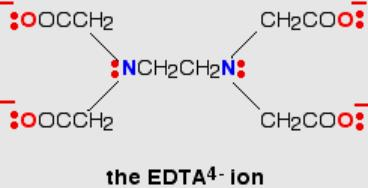*Answer can only contain numeric values
QUESTION: 21

The oxidation state of the central metai in the complex ion [Fe(EDTA)]- .

Solution:

In [Fe(EDTA)]-, Let the oxidation state is x then
x - 4 = - 1
⇒ x = 4 - 1 = 3

*Answer can only contain numeric values
QUESTION: 22

The complex potassium dicyanodioxalatonickelate (II) in solution produce....... ions.

Solution:

The structure of potassium dicyanodio xalatonickelate (II) is
K4[Ni(CN)2(ox)2].
K4[Ni(CN)2(ox)2] →  4K+ [Ni(CN)2(ox)2]-
This produce 5 ions in solution.

*Answer can only contain numeric values
QUESTION: 23

Number of EDTA molecules required to form an octahedral complex.

Solution:

EDTA is hexadentate ligand hence, its coordination number is 6.

QUESTION: 24

IUPAC name of [Pt(NH3)2Cl(NO2)] is

Solution:

Ans: c

Explanation:Pt(NH3)2Cl(NO2)

m − 2 = 0

m = +2

(NH3)2 ⇒ Diammine

Cl ⇒ Chlorido

NO2 ⇒ Nitrito-N.

So, IUPAC NAME: diamminechloridonitrito-N-platinum(II)

QUESTION: 25

Statement Type

Direction (Q. No. 25) This section is based on Statement I and Statement II. Select the correct answer from the codes given below.

Q.

Statement l : IUPAC name of the complex [(NH3)5—Cr—OH—Cr (NH3)5]CI5 is pentammine chromium-μ-hydroxopentammine chromium (III) chloride.

Statement II : IUPAC name of the complex [Ni(dmg)2] is bis (dimethylglyoximato) nickel (III).

Solution:

[Ni(dmg)2] : bis (dimethylglyoximato) nickel (II)